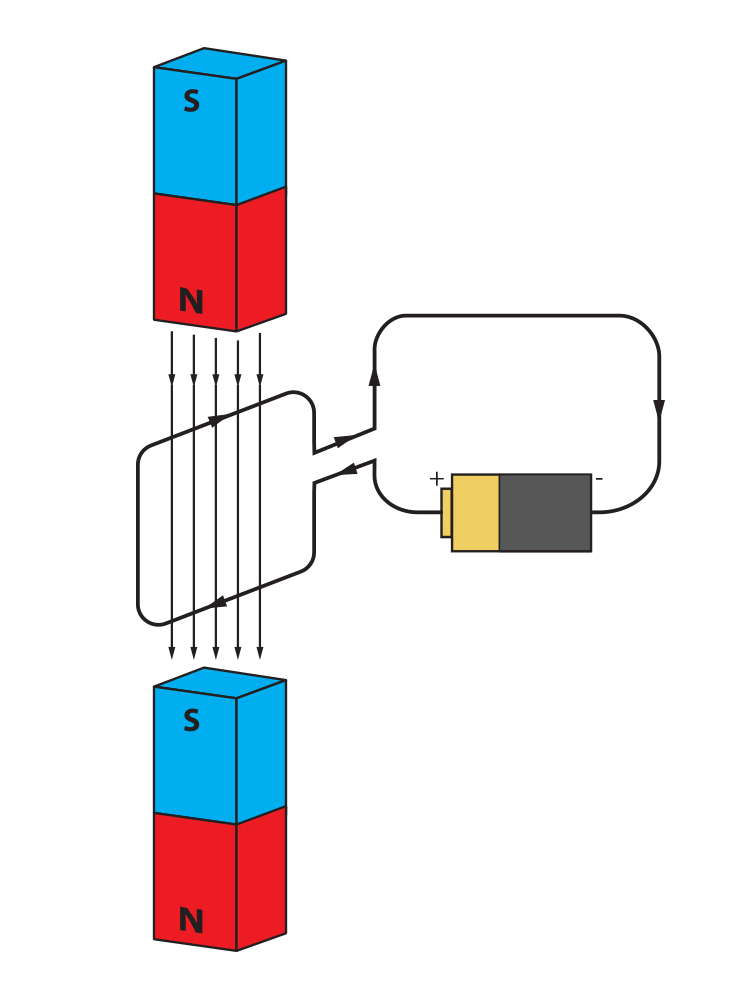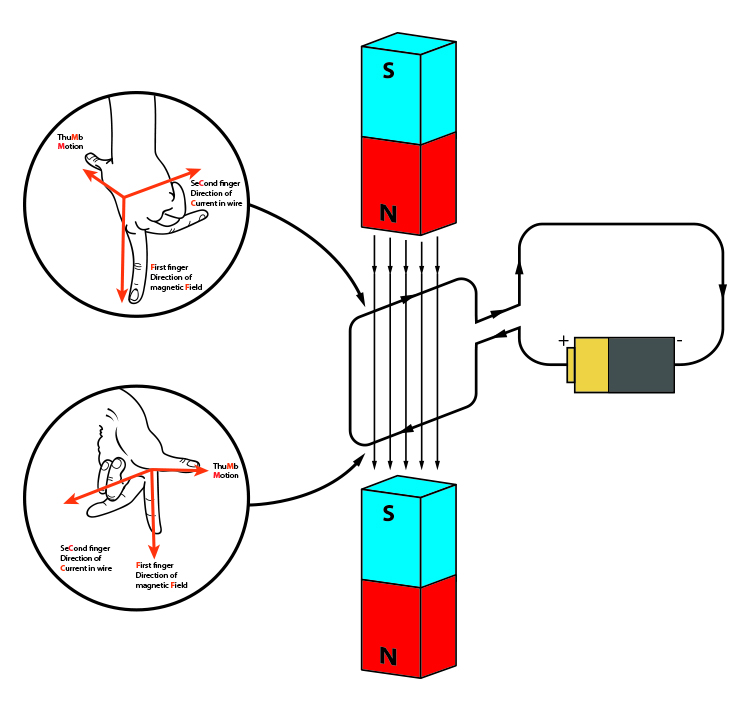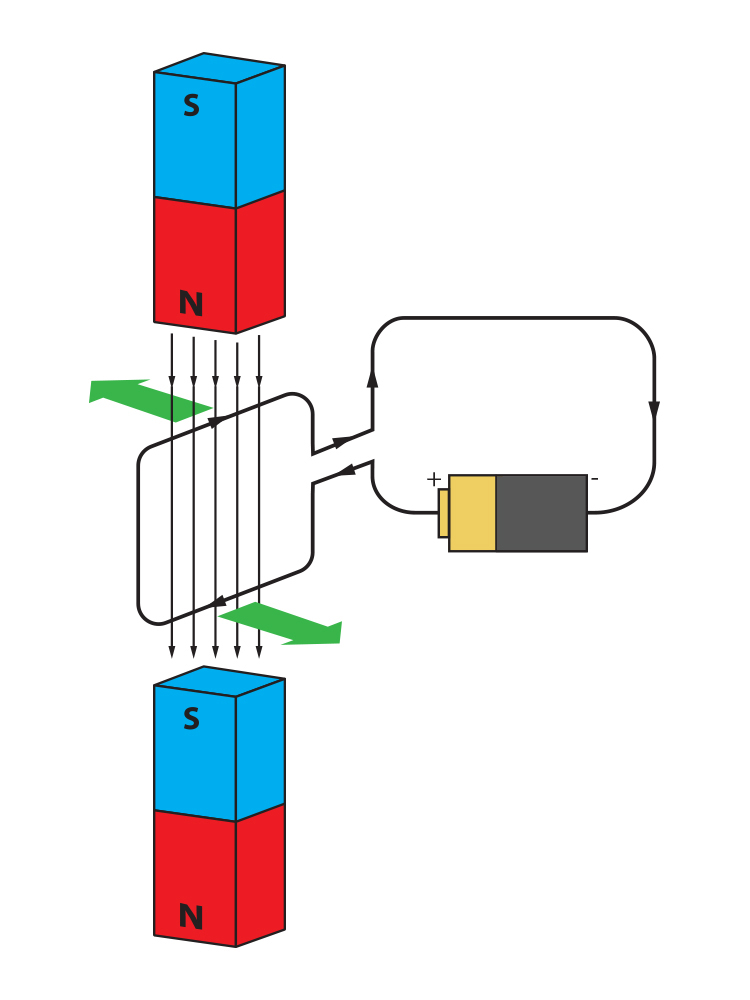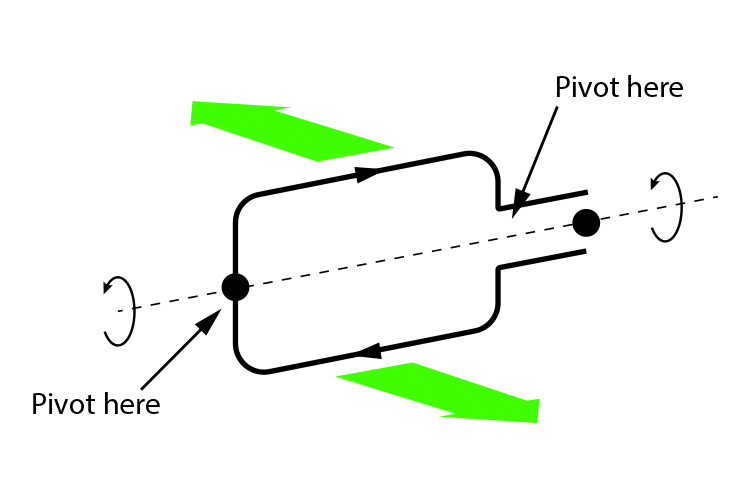# An electric coil between two magnets

We can see from the diagram below that within the magnetic field, the current travelling along the loop of wire is moving to the right at the top of the loop and to the left at the bottom - ie, opposite directions.If we use Fleming's left-hand rule, which is used when electricity is already being passed through a wire, we can determine the direction of the forces on each of the wires.The forces of motion on the wires can be shown as follows:If we could pivot this coiled wire we would get a turning motion.The coil would start to spin